# ISEE Upper Level Math : How to find the length of the side of a square

## Example Questions

### Example Question #1 : How To Find The Length Of The Side Of A Square

The area of a square is 169 square inches. What is the length of one side (in the diagram below)?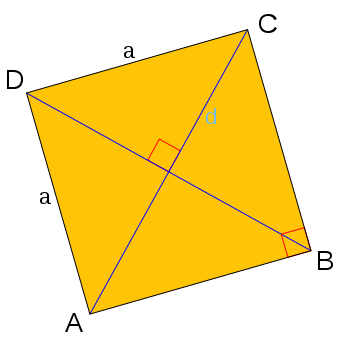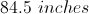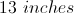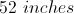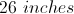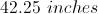Explanation:

Area of a quadrilateral is found by length times width. In a square, these are the same, so the length of sideis a number that, when multiplied by itself is equal to 169.

In other words, take the square root of 169 to find the length of.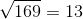### Example Question #2 : How To Find The Length Of The Side Of A Square

If the perimeter of a square is 36in, what is the length of one side?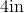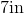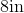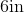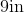Explanation:

To find the perimeter of a square, we will use the following formula: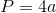where a is the length of any side of the square.  Because a square has 4 equal sides, we can use any side in the formula.  To find the length of any side, we will solve for a.

Now, we know the perimeter of the square is 36in.  So, we will substitute.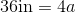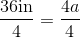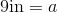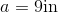Therefore, the length of one side of the square is 9in.

### Example Question #3 : How To Find The Length Of The Side Of A Square

Your new friend has a very small, square-shaped dorm room. She tells you that it is only 225 square feet. Assuming this is true, what is the length of one side of her room?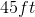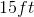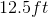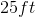Explanation:

Your new friend has a very small, square-shaped dorm room. She tells you that it is only 225 square feet. Assuming this is true, what is the length of one side of her room?

Let's begin with our formula for the area of a square: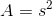where s is our side length and A is our area.

With this formula, we can solve for our side length by plugging in our area and square rooting both sides.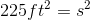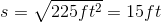### Example Question #4 : How To Find The Length Of The Side Of A Square

A square has a perimeter of 60cm.  Find the length of one side.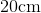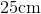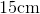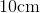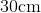Explanation:

A square has 4 equal sides.  The formula to find perimeter of a square is:where a is the length of any side.  Now, to find the length of one side, we will solve for a.

We know the perimeter of the square is 60cm.  So, we can substitute and solve for a.  We get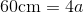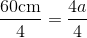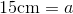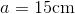Therefore, the length of one side of the square is 15cm.